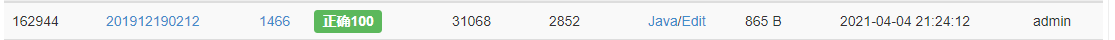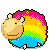# [蓝桥杯2019初赛]等差数列，数学老师给小明出了一道等差数列求和的题目。但是粗心的小明忘记了一部分的数列，只记得其中N 个整数。 现在给出这N 个整数，小明想知道包含这N 个整数的最短的等差数列有几

## [蓝桥杯2019初赛]等差数列java简单方法。完美AC

2<=N<=100000,0<=Ai<=10^9

5
2 6 4 10 20

10

AC代码：

``````import java.util.Arrays;
import java.util.Scanner;

public class Main {
public static long diff;
public static void main(String[] args) {

Scanner scanner=new Scanner(System.in);
int n=scanner.nextInt();
int arr[]=new int[n];
for (int i = 0; i < n; i++) {
arr[i]=scanner.nextInt();
}
Arrays.sort(arr);

int diff=0;
for (int i = 0; i <n-1; i++) {
diff=gcd(diff,arr[i+1]-arr[i]);
}

if (diff==0){
System.out.println(n);
}
else {
long count=0;
System.out.println((arr[n-1]-arr)/diff+1);
}
}
public static int gcd(int a,int b) {//欧几里得算法（辗转相除法）
return b!=0?gcd(b, a%b):a;//求两个正整数的最大公约数
}

}

``````